# ANSYS扳手可靠性分析${\sigma _{\max }} \ge \sigma _{s }$

$G(x) = {\sigma _s} – {\sigma _{\max }}$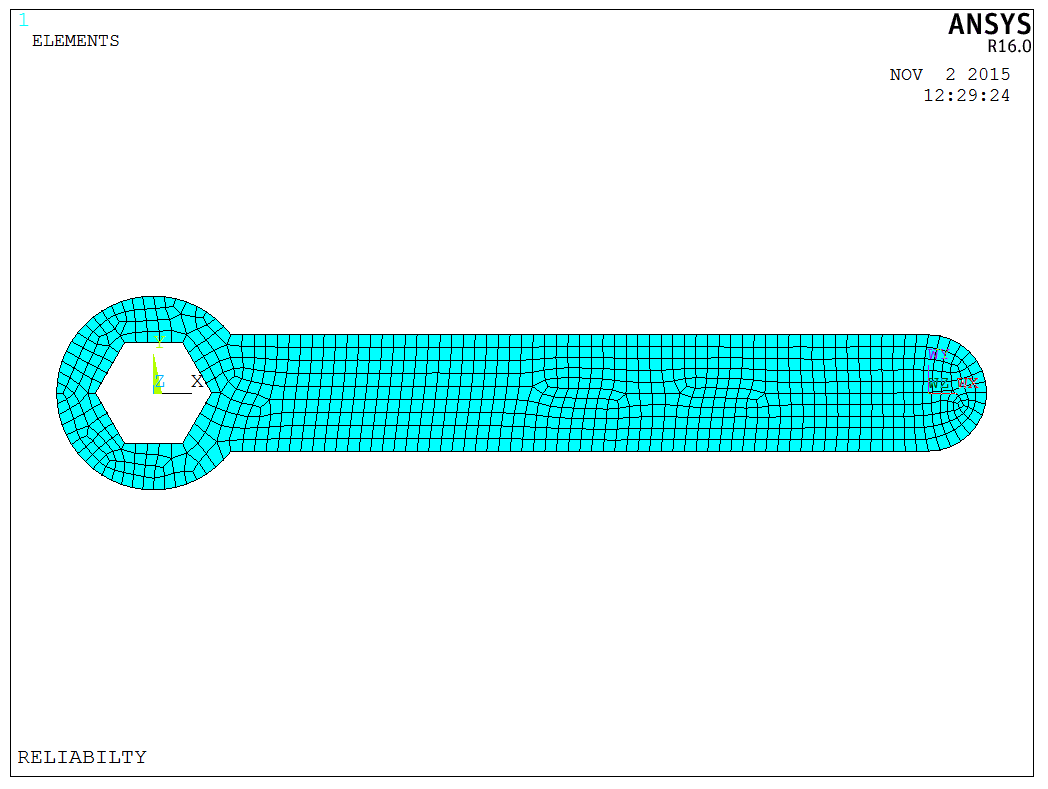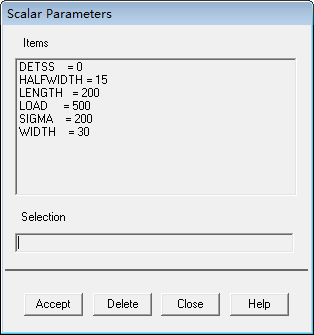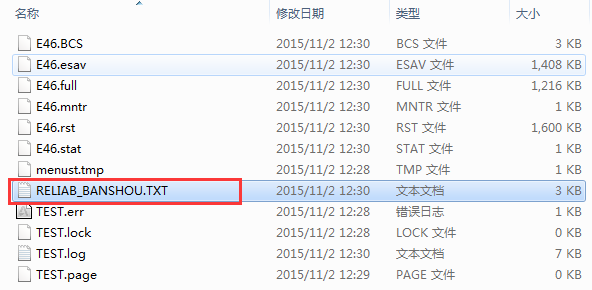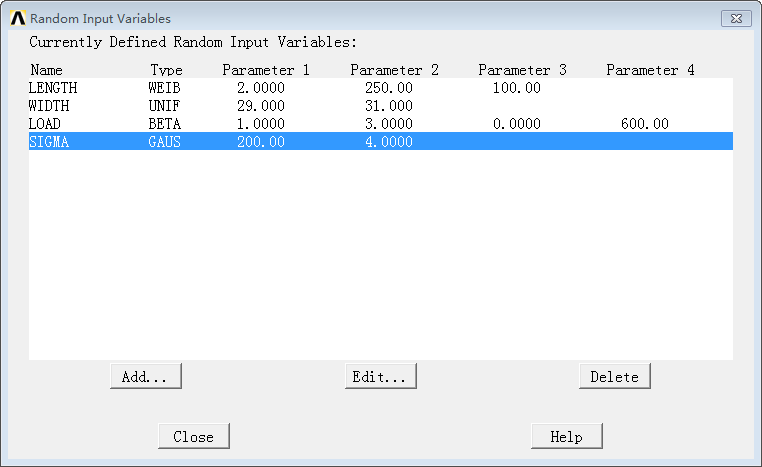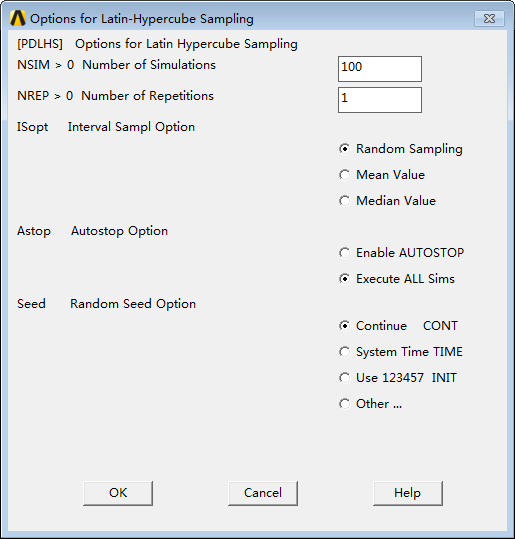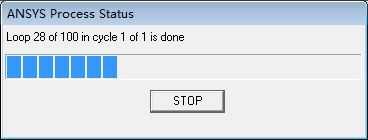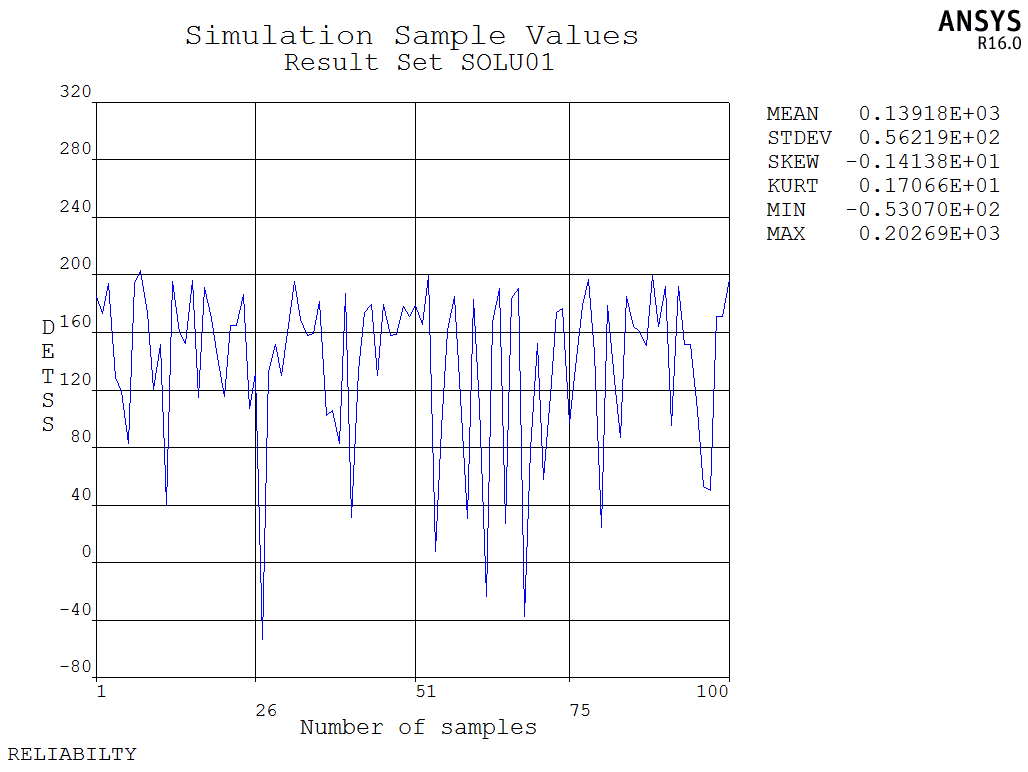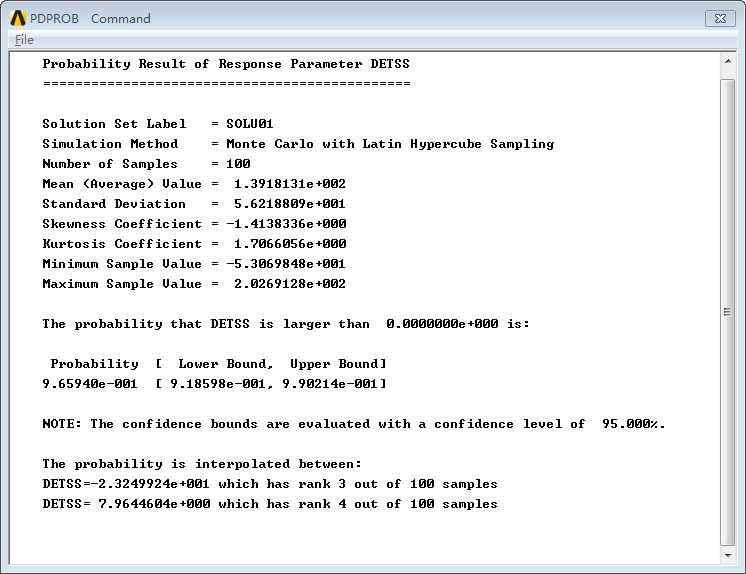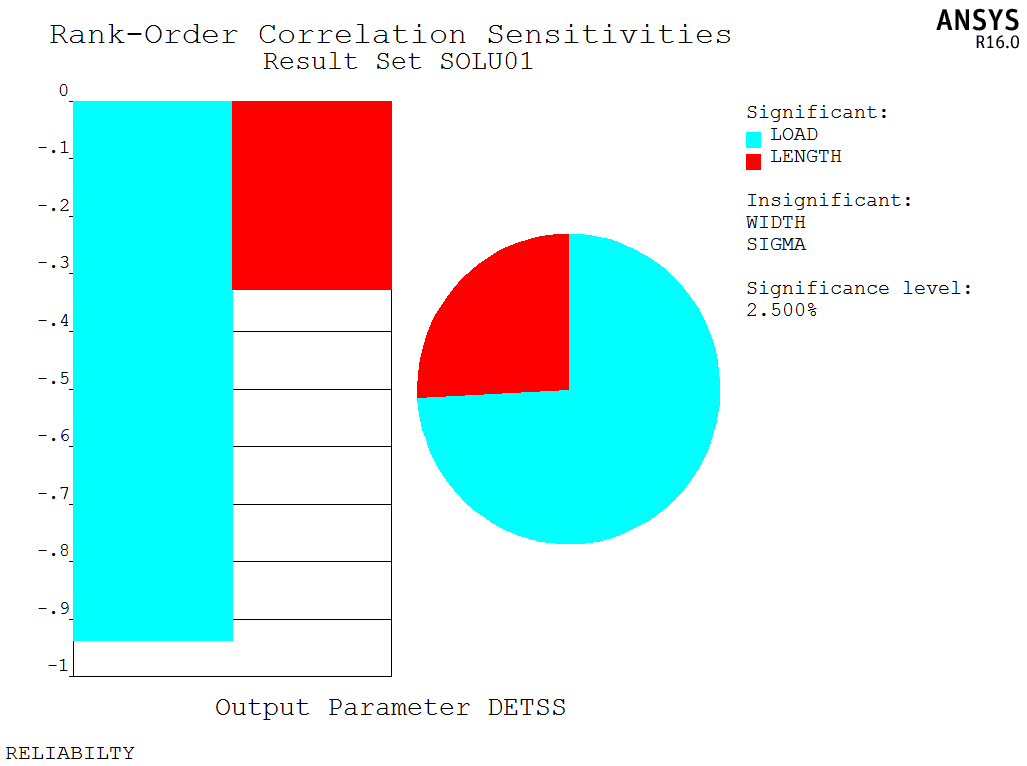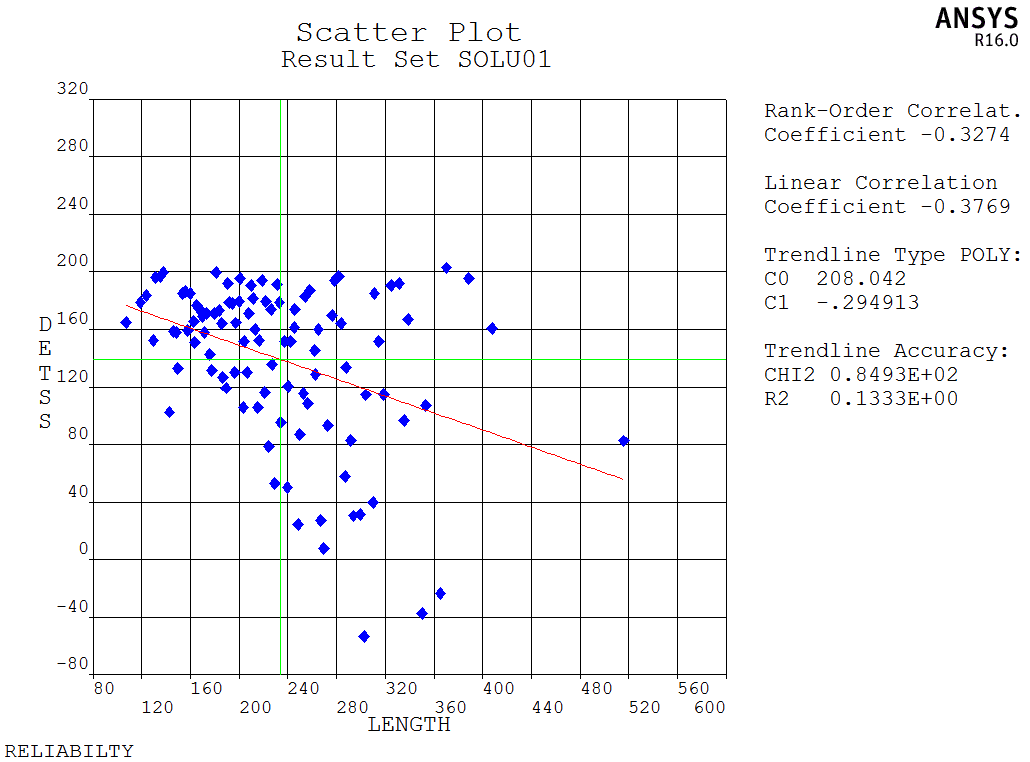APDL代码如下所示：